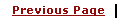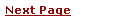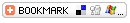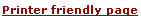C - Using ConstantsLearning C
C Function References
C Useful Resources# C - Using ConstantsA C constant is usually just the written version of a number. For example 1, 0, 5.73, 12.5e9. We can specify our constants in octal or hexadecimal, or force them to be treated as long integers.

• Octal constants are written with a leading zero - 015.
• Long constants are written with a trailing L - 890L.
Character constants are usually just the character enclosed in single quotes; 'a', 'b', 'c'. Some characters can't be represented in this way, so we use a 2 character sequence as follows.
 ```'\n' newline '\t' tab '\\' backslash '\'' single quote '\0' null ( Usedautomatically to terminate character string ) ```

In addition, a required bit pattern can be specified using its octal equivalent.

'\044' produces bit pattern 00100100.

Character constants are rarely used, since string constants are more convenient. A string constant is surrounded by double quotes eg "Brian and Dennis". The string is actually stored as an array of characters. The null character '\0' is automatically placed at the end of such a string to act as a string terminator.

A character is a different type to a single character string. This is important poing to note.

# Defining Constants

ANSI C allows you to declare constants. When you declare a constant it is a bit like a variable declaration except the value cannot be changed.

The const keyword is to declare a constant, as shown below:

 ```int const a = 1; const int a =2; ```

Note:

• You can declare the const before or after the type. Choose one an stick to it.
• It is usual to initialise a const with a value as it cannot get a value any other way.

The preprocessor #define is another more flexible (see Preprocessor Chapters) method to define constants in a program.

 ```#define TRUE 1 #define FALSE 0 #define NAME_SIZE 20 ```

Here TRUE, FALSE and NAME_SIZE are constant

You frequently see const declaration in function parameters. This says simply that the function is not going to change the value of the parameter.

The following function definition used concepts we have not met (see chapters on functions, strings, pointers, and standard libraries) but for completenes of this section it is is included here:

 ```void strcpy(char *buffer, char const *string) ```

# The enum Data type

enum is the abbreviation for ENUMERATE, and we can use this keyword to declare and initialize a sequence of integer constants. Here's an example:

 ```enum colors {RED, YELLOW, GREEN, BLUE}; ```

I've made the constant names uppercase, but you can name them which ever way you want.

Here, colors is the name given to the set of constants - the name is optional. Now, if you don't assign a value to a constant, the default value for the first one in the list - RED in our case, has the value of 0. The rest of the undefined constants have a value 1 more than the one before, so in our case, YELLOW is 1, GREEN is 2 and BLUE is 3.

But you can assign values if you wanted to:

 ```enum colors {RED=1, YELLOW, GREEN=6, BLUE }; ```

Now RED=1, YELLOW=2, GREEN=6 and BLUE=7.

The main advantage of enum is that if you don't initialize your constants, each one would have a unique value. The first would be zero and the rest would then count upwards.

You can name your constants in a weird order if you really wanted...

 ```#include int main() { enum {RED=5, YELLOW, GREEN=4, BLUE}; printf("RED = %d\n", RED); printf("YELLOW = %d\n", YELLOW); printf("GREEN = %d\n", GREEN); printf("BLUE = %d\n", BLUE); return 0; } This will produce following results RED = 5 YELLOW = 6 GREEN = 4 BLUE = 5 ```